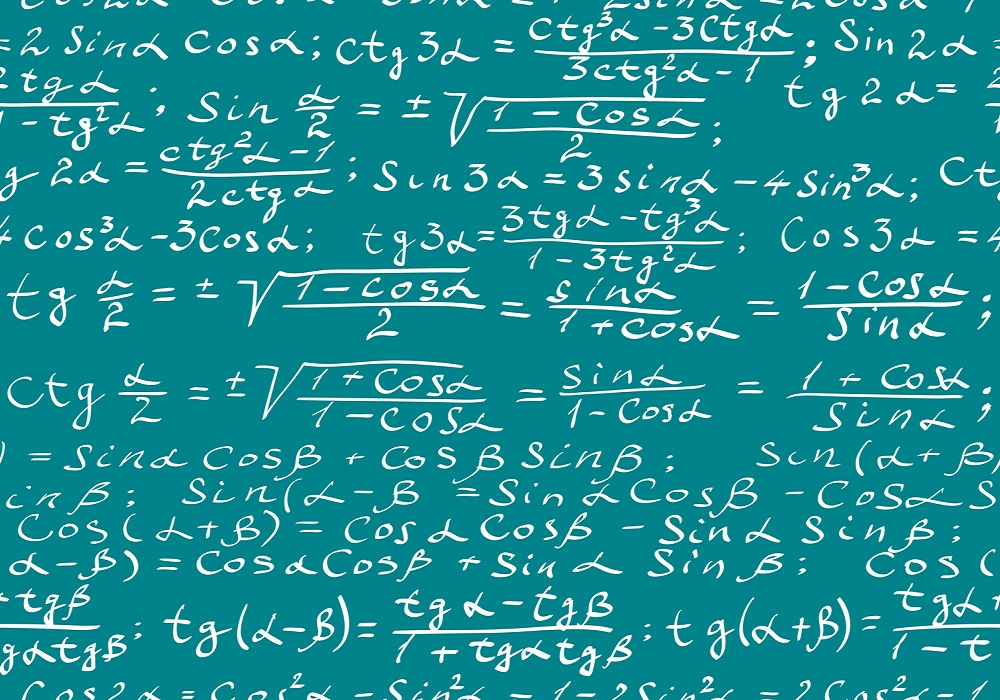# The Math in ML: Cosine SimilarityIn Machine Learning, we frequently express the similarity between two vectors as cosine similarity. What exactly does that mean? What has ‘cosine’ got to do with similarity? Well, there is Math in ML and cosine similarity between vectors has everything to do with Math. Let us refresh what is a vector, and then we come to what is the cosine between two vectors. What is a Vector? A vector is a column of numbers enclosed in brackets, like this. As a special case, a two dimensional vector is a column of two numbers enclosed in brackets, like this. When you…...